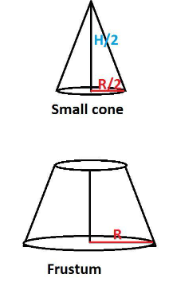Courses
Courses for Kids
Free study material
Free LIVE classes
MoreLIVE
Join Vedantu’s FREE Mastercalss

# The height of a cone is 10cm. The cone is divided into two parts by drawing a plane through the midpoint of the axis of the cone, parallel to the base. Compare the volume of the two parts.A.1 : 7B.2 : 9C.3 : 11D.3 : 5Verified
364.2k+ views
Hint: volume of a cone with radius r and height h is equal to $\dfrac{1}{3}\pi {r^2}h.$

Let the height of the given cone be h cm.
After dividing in two parts, we get
Resulting frustum has height half of the original cone and radius at the top of the frustum equal to half of the base radius of the cone.
Frustum of the cone with radius, R=10 cm and radius r = 5 cm, height = $\dfrac{h}{2}$ cm
A smaller cone of radius, r =(R/2) = 5 cm and height = $\dfrac{h}{2}$ cm
$\therefore$ Ratio of volumes = (volume of smaller cone) / (volume of frustum of cone)
$= \dfrac{{\dfrac{1}{3}\pi {r^2}\left( {\dfrac{h}{2}} \right)}}{{\dfrac{1}{3}\pi \left( {\dfrac{h}{2}} \right)\left[ {{R^2} + {r^2} + Rr} \right]}}$
$= \dfrac{{5 \times 5}}{{\left[ {{{10}^2} + {5^2} + 10 \times 5} \right]}} = \dfrac{{25}}{{175}}$
$= \dfrac{1}{7}$
Therefore, 1:7 is the required ratio.
Note: we have to understand the given problem clearly. If we imagine the problem in geometric structure it is easy to solve. When we divide a cone as mentioned in the problem it looks similar to the below figure.Last updated date: 30th Sep 2023
Total views: 364.2k
Views today: 10.64k